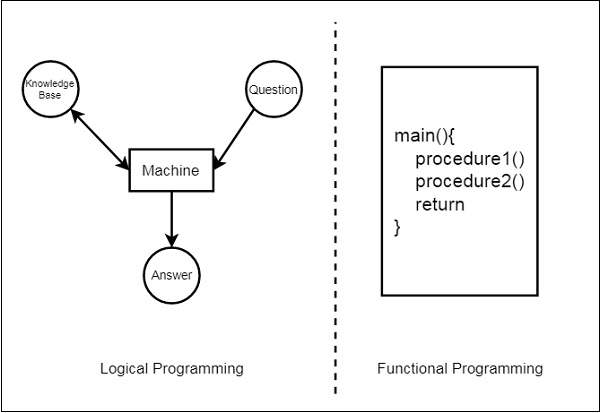# Prolog - Introduction

Prolog as the name itself suggests, is the short form of LOGical PROgramming. It is a logical and declarative programming language. Before diving deep into the concepts of Prolog, let us first understand what exactly logical programming is.

Logic Programming is one of the Computer Programming Paradigm, in which the program statements express the facts and rules about different problems within a system of formal logic. Here, the rules are written in the form of logical clauses, where head and body are present. For example, H is head and B1, B2, B3 are the elements of the body. Now if we state that “H is true, when B1, B2, B3 all are true”, this is a rule. On the other hand, facts are like the rules, but without any body. So, an example of fact is “H is true”.

Some logic programming languages like Datalog or ASP (Answer Set Programming) are known as purely declarative languages. These languages allow statements about what the program should accomplish. There is no such step-by-step instruction on how to perform the task. However, other languages like Prolog, have declarative and also imperative properties. This may also include procedural statements like “To solve the problem H, perform B1, B2 and B3”.

Some logic programming languages are given below −

• ALF (algebraic logic functional programming language).

• CycL

• Datalog

• FuzzyCLIPS

• Janus

• Parlog

• Prolog

• Prolog++

• ROOP

## Logic and Functional Programming

We will discuss about the differences between Logic programming and the traditional functional programming languages. We can illustrate these two using the below diagram −From this illustration, we can see that in Functional Programming, we have to define the procedures, and the rule how the procedures work. These procedures work step by step to solve one specific problem based on the algorithm. On the other hand, for the Logic Programming, we will provide knowledge base. Using this knowledge base, the machine can find answers to the given questions, which is totally different from functional programming.

In functional programming, we have to mention how one problem can be solved, but in logic programming we have to specify for which problem we actually want the solution. Then the logic programming automatically finds a suitable solution that will help us solve that specific problem.

Now let us see some more differences below −

Functional Programming Logic Programming
Functional Programming follows the Von-Neumann Architecture, or uses the sequential steps. Logic Programming uses abstract model, or deals with objects and their relationships.
The syntax is actually the sequence of statements like (a, s, I). The syntax is basically the logic formulae (Horn Clauses).
The computation takes part by executing the statements sequentially. It computes by deducting the clauses.
Logic and controls are mixed together. Logics and controls can be separated.

## What is Prolog?

Prolog or PROgramming in LOGics is a logical and declarative programming language. It is one major example of the fourth generation language that supports the declarative programming paradigm. This is particularly suitable for programs that involve symbolic or non-numeric computation. This is the main reason to use Prolog as the programming language in Artificial Intelligence, where symbol manipulation and inference manipulation are the fundamental tasks.

In Prolog, we need not mention the way how one problem can be solved, we just need to mention what the problem is, so that Prolog automatically solves it. However, in Prolog we are supposed to give clues as the solution method.

Prolog language basically has three different elements −

Facts − The fact is predicate that is true, for example, if we say, “Tom is the son of Jack”, then this is a fact.

Rules − Rules are extinctions of facts that contain conditional clauses. To satisfy a rule these conditions should be met. For example, if we define a rule as −

```grandfather(X, Y) :- father(X, Z), parent(Z, Y)
```

This implies that for X to be the grandfather of Y, Z should be a parent of Y and X should be father of Z.

Questions − And to run a prolog program, we need some questions, and those questions can be answered by the given facts and rules.

## History of Prolog

The heritage of prolog includes the research on theorem provers and some other automated deduction system that were developed in 1960s and 1970s. The Inference mechanism of the Prolog is based on Robinson’s Resolution Principle, that was proposed in 1965, and Answer extracting mechanism by Green (1968). These ideas came together forcefully with the advent of linear resolution procedures.

The explicit goal-directed linear resolution procedures, gave impetus to the development of a general purpose logic programming system. The first Prolog was the Marseille Prolog based on the work by Colmerauer in the year 1970. The manual of this Marseille Prolog interpreter (Roussel, 1975) was the first detailed description of the Prolog language.

Prolog is also considered as a fourth generation programming language supporting the declarative programming paradigm. The well-known Japanese Fifth-Generation Computer Project, that was announced in 1981, adopted Prolog as a development language, and thereby grabbed considerable attention on the language and its capabilities.

## Some Applications of Prolog

Prolog is used in various domains. It plays a vital role in automation system. Following are some other important fields where Prolog is used −

• Intelligent Database Retrieval

• Natural Language Understanding

• Specification Language

• Machine Learning

• Robot Planning

• Automation System

• Problem Solving